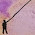## 21 Jun 2011

### Pendulum Waves - Mathematical Description cont.

This is the second of a three part post on mathematically explaining the pendulum waves apparatus (below). The first part is located here.

We'll start off from where we ended last time.
We had two possible equations that we could use to continue our derivation with:

$y(x,t)=A\cos\left(k_{0}x+\omega(x)t\right)$$y(x,t)=A\cos\left(k(t)x+\omega_{0}t\right).$

Let's use the first of the two equations above.

Since we start the pendulum waves by setting all the pendula to maximum amplitude, we have $y\left(x,0\right)=A=A\cos\left(k_{0}x+\omega\left(0\right)\cdot0\right)$$A=A\cos\left(k_{0}x\right)\implies k_{0}=0.$
Let's figure out what $\omega(x)$ is. We'll forget about $\omega$ as a function of $x$ for a moment and look at $\omega$ as a function of the n-th pendulum instead.

Recall that the total time of the dance is $\Gamma$ during which the longest pendulum performs $N$ oscillations. So the period of the longest pendulum is $T=\frac{\Gamma}{N}$. OK, so we have the period of one pendulum now. How do we figure out the gabajillion other pendulums on the thing? Well, we also know that as the pendulums get shorter, their number of oscilations increases. More specifically, if the longest pendulum has 51 oscillations, then its shorter neighbour will have 52 oscillations, its shorter neighbour will have 53 oscillations, and so forth. So letting $n$ represent the n-th pendulum (counting from 0), the period of any pendulum on the apparatus is $T=\frac{\Gamma}{N+n}$.

To express $\omega$ as a function of $x$, note that the x-position of the pendulum is $x=nd$ (so $n=\frac{x}{d}$ where $d$ is the common spacing between adjacent pendulums. We're almost done now. Now we can see that: $\omega(x)=\frac{2\pi}{T}=\frac{2\pi}{\frac{\Gamma}{N+\frac{x}{d}}}$ $\omega(x)=\frac{2\pi \left(N+\frac{x}{d}\right)}{\Gamma}=\frac{2\pi \left(Nd+x\right)}{\Gamma d}$
Therefore, our complete wave equation is: $y(x,t)=A\cos\left(\frac{2\pi \left(Nd+x\right)}{\Gamma d}t\right)$
As a note of interest, we can rewrite the equation above as
$y(x,t)=A\cos\left(\frac{2\pi t}{\Gamma d}x + \frac{2\pi N}{\Gamma}t\right)$ which corresponds to $y(x,t)=A\cos\left(k(t)x+\omega_{0}t\right).$

And we are done! That's the equation describing all of the waves you see in the video above.

Now that we are finished, we can take a nice break and prepare ourselves for the next post, where I'll discuss the properties of the equation we've just found.

1.hey this is a very good explanation of how to build a pendulum but i still dont get where the 51 came from can yu explain in simple words

1.51 was just the number of oscillations the people in the video chose for their pendulum. You could change it if you want, but the wave effect of the pendulum would become quicker or slower depending on how you changed the number of oscillations.

2.how do yu figure out the oscillation

1.You can count it from the video, or read the description here.

3.would the angle that yu pull the pendulum be a factor

1.The frequency will be affected because the small angle approximation used in the calculations will be invalid. However, the pendulum wave effect will be the same if not more awesome.

4.These explanations are really fantastic and easy to grasp. I'm a high school sophomore and my native language isn't English but I understood it very well. Thanks very very much!

1.Thank you! It's comments like these that keep me going!

5.Thank you for your excellent post Paul. I've been looking for a nice explanation of the pendulum wave formula. I will link to your page on my site, so hopefully you'll get some additional visitors.

Best of luck on figuring out what you want to do in life. I'll give you a hint though--I don't think any of us completely figures that one out. I think the best thing we can do is to follow our heart and do the things that we enjoy that can be of service to others.

1.Thanks, Chris! I checked out your blog -- it's awesome!

The advice is also extremely helpful. Too bad it seems like time is always running short. There are a thousand things I'd like to try, and if I had time, I'd try them all.

Cheers,
Paul

6.Where do I find the first description because I don't see anything obvious in your deductions.
I assume that it's correct - because I also assume that this is probably some standard motion example from 100 math courses ... but I don't see what it is that allows you to make assumptions.
Why should we forget that ω is a function of x for a moment, and look at ω as a function of the n-th pendulum instead. Is this something obvious or just convenient?
Nevermind, where is the first part posted on this pendulum wave study?

7.Hi George,

Sorry for the late reply! The first part of my blog post is located at http://hippomath.blogspot.com/2011/06/pendulum-waves-mathematical-description.html.

Cheers,
Paul

8.Drew Kesler (Newark Academy)14 September 2013 at 13:55

Hi Paul, longtime fan here! I hope you know how many physics teachers depend on you! I've built a crummy version of this, with just a constant change in length to the next pendulum. Now that I found your posts I've just learned how I can make it so much better. Thanks a whole lot.

1.Thanks Drew, I appreciate the support!

9.Thanks Paul, what is the "d" value?

1.Hi Anon,

The d is the separation between each pendulum bob.

Cheers,
Paul

10.Hello Paul Liu! I am dave from South Korea!
and I made my own pendulum waves machine
http://youtu.be/fq5bnmHuA7E
and I hv to display this machine and poster about it.
So can i use some of pics in here for my poster?

Thx you for ur kind description! it helps me a lot!

1.Hi David,

Your pendulum wave machine looks awesome! You are free to use any pictures here if you want.

Cheers,
Paul

11.Hello Paul Liu! I'm interested in making my own pendulum wave and your explanation is really helpful. I was wondering what this experiment proves?